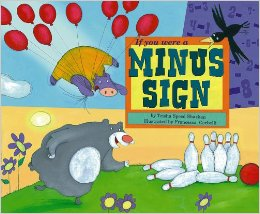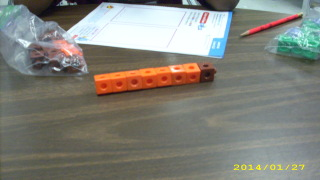# Missing Numbers

24 teachers like this lesson
Print Lesson

## Objective

SWBAT use related facts to determine unknown numbers.

#### Big Idea

What's missing? In this lesson students will learn to find the missing number in a given math sentence.

## Activating Strategy

10 minutes

I like to start this lesson by reading the book “If you were a minus signI like to use this book because it demonstrates the many ways we subtract even in our daily lives.

I will then write an addition word problem on the board/chart paper for example: John has 8 baseball cards and 7 football cards.  How many sports cards does he have in all?  I like to guide the discussion about how to solve the problem using different strategies.  For example:

• How can you solve the problem? (I can make a model; or I can draw a picture)

Symbols such as +, –, and = convey clear mathematical ideas. Each symbol has a precise meaning (MP6); when one symbol is used with another, such as 5 + 1 = 6, the combination of symbols conveys a more complex meaning than the individual symbols.

Children generally build meaning for the basic operations in this order: through modeling (concrete), through pictorial representations (linking), and through abstract thinking (symbolic). Children often have the most difficulty with this last step.

Before children can gain fluency with using symbols to communicate precise mathematical ideas, they must understand the relationship among the operations and among the different symbols.

Help children connect the symbols +, –, and = to the relationship between addition and subtraction. Write 10  3 = ___ on the board, and guide the discussion:

• Do you need to add or subtract? How do you know? (Subtract. There is a minus sign.)

• What does the line mean? (The difference is missing.)

Demonstrate how to use addition to find the difference. Write 3 + ___ = 10 on the board.

• How is this problem like the first? (It has the same numbers, an equal sign, and a box for the missing number.)

• How is it different? (It has a plus sign instead of a minus sign. The line is in a different place.)

• Is the missing number the same in both problems? Explain.  (Yes. The missing number is 7. 7 is the difference in the subtraction problem. 7 is the missing addend in the addition problem.)

## Teaching Strategies

15 minutes

Children may ask how to figure out the missing number that completes the addition or subtraction sentence, the idea is to review the strategies of related facts, the commutative property of addition or using addition to check subtraction.

Make sure that children understand that missing addends may be found using different methods, and encourage them to use the relationship between addition and subtraction to find the missing numbers.

To begin the lesson (Missing Numbers), I pose the following problem.  “Calvin has 7 toy cars that are red.  He has some blue cars too.  He has 10 toy cars.  How many blue toy cars does Calvin have?”  To help children see the concrete aspect of the problem, I generally have them model the problem with connecting cubes.  I guide the discussion on how to solve the problem using these questions:

• How many toy cars are in the whole group? (10)
• What are the two parts of our group? (7 red cars, and some blue cars)
• Will we solve the problem using addition or subtraction?  (subtraction: I can subtract 7 from 10 to get 3; addition: I can count on from 7 to 10 to get 3)

I then model another addition and subtraction sentence to show how to relate the two sentences.  For example:

• 8 + _____ = 11                                              11 – 8 = _____

Using a model (connecting cubes) I like to work through the problem with the students.  The conversation goes something like this:

• How are the two facts the same? (they both have 8 and 11)
• How are they different? (one is addition, and one is subtraction)
• How many cubes do we need to add to 8 to get a total of 11? (3)
• How many cubes are left after you take 8 from 11? (3)
• How are these facts related? (the subtraction fact undoes the addition fact; or both sentences use the same numbers)

## Independent Practice

30 minutes

Once the students understand the concept of determining the unknown number, I hand out Missing numbers worksheet for them to complete.

Here is a picture of a student using connecting cubes to help model their problem.  Allowing students to use models to solve for missing numbers is important for first graders.For those struggling students, I write several addition problems on the board/chart paper with numbers missing from different positions in the sentence.  For example, you might have some missing the sum, some missing the first addend, and some missing the second addend.  Ask children to use connecting cubes to model the problems to discover the missing numbers.  Have children write the related subtraction sentence that helps them find a missing addend.

For example:

6 + ____ = 10

Children would use 6 red cubes and then add blue cubes to their cube train until they reach 10 cubes.  This allows for a concrete model representation of the addition sentence, which allows for them to visualize how to solve the problem.

## Closing/Summarizing

5 minutes

To close out this lesson students get their math journals and have them use pictures, or numbers to show how to find the missing numbers for:

8 + ______ = 17 and 17 – 8 = ______.